# Use a protractor to measure angles

👁 38
statistics

How to use protractor to measure angles

Questions #: 15
Time: 10 minutes
Pass Score: 80.0%
Style
Mode

POINTS (1)

POINTS (1)

POINTS (1)

#### An angle measure of 1° meaning

An angle measure of 1° (read as “one degree”) means that one side of an angle is rotated ________ of a complete revolution about the vertex from the other side of the angle

POINTS (1)

#### Explanation

The measure of ∠ ABC , shown below, is 1°. We can write this in symbols as m(∠ ABC) = 1°POINTS (1)

#### An angle measure equivalency

• An angle measure of 90° is equivalent to (1)• An angle measure of 180° is equivalent to (2)• An angle measure of 270° is equivalent to (3)drag and drop the selected option to the right place or type it instead
$\frac{1}{4}$
$\frac{3}{4}$
$\frac{1}{2}$
POINTS (1)

#### Match angles to the diagrams

•(1)•(2)•(3)drag and drop the selected option to the right place or type it instead
m(∠FED) = 270°
m(∠FED) = 180°
m(∠FED) = 90°
m(∠FED) = 360°
POINTS (1)

POINTS (1)

#### Write the correct measure of angles in the below table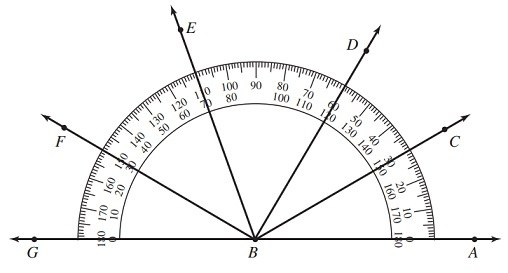• ABC = °
• ABD = °
• ABE = °
• ABF = °
• ABG = °
• GBF = °
• GBC = °
POINTS (1)

Missing
POINTS (1)

#### Classifying angles

Types of angles
• (1): Angles whose measures are greater than 0° but less than 90°.
• (2): Angles whose measures are 90°.
• (3): Angles whose measures are greater than 90° but less than 180°.
• (4): Angles whose measures are 180°
drag and drop the selected option to the right place or type it instead
Straight angles
Acute angles
Obtuse angles
Right angles
POINTS (1)

#### Match angles with diagrams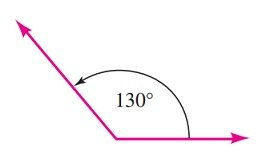(1)(2)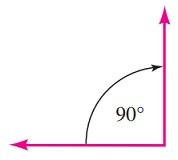(3)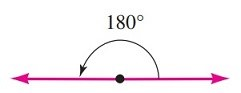(4)drag and drop the selected option to the right place or type it instead
Acute angle
Obtuse angle
straight angle
Right angle
POINTS (1)

#### Right angle symbol

Choose the symbol which is commonly drawn in a right angle

POINTS (1)

#### Classify each angle in the figure as an acute angle, a right angle, an obtuse angle, or a straight angle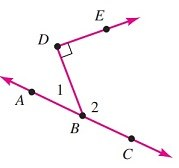Since m(∠1) is an (1)angle.

Since m(∠2) is an (2)angle.

Since m(∠BDE) is a (3)angle.

Since m(∠ABC) is a (4)angle

drag and drop the selected option to the right place or type it instead
straight
acute
right
obtuse
POINTS (1)

#### Classify the following angles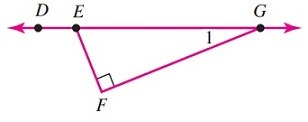• ∠ DEG is (1)angle
• ∠ DEF is (2)angle
• EFG is (3)angle
• ∠ EGF is (4)angle
• ∠ GEF is (5)angle

drag and drop the selected option to the right place or type it instead
obtuse
straight
acute
right
POINTS (1)